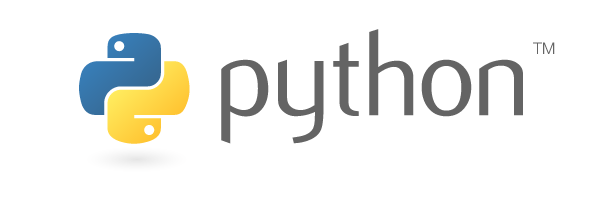Ananta Almost a Computer Engineer, author of Go Woogle, I write about tech and tutorials.

# Data Types in Python## Python Fast Track - 1

If you are new to this coding thing first line of any coding is almost always the legendary “hello world!”.

Let’s start by printing hello world in python shell if you don’t have python installed or don’t know how to start a python shell in terminal click here for basic terminal skills and here for python installation

``````1
print("Hello World!")
``````

above line simply prints `Hello World!`

## Data Types

A “Data Type” is simply a classification of information. The data type of an object dictates the different things that we can do to it and use it for.

In Python there are many different data types but the ones we are going to talk about now are the following:

• Integer: In python integers are represented by the keyword “int”. Int’s are any whole number (negative or positive).

• Float: In python floats are represented by the keyword “float”. Floats’s are any decimal number (negative or positive).

• String: In python strings are represented by the keyword “str”. Str’s are any anything surrounded by double quotes (“im a string”) or single quotes (‘i am also a string’).

• Boolean: In python boolean’s are represented by the keyword “bool”. Bools are either True or False (Capitals Matter!).

Some Examples:

``````1
2
3
4
int: 1, 3, 5, 9, -3, 0
float: 1.0, 0.9, -0.7, 5.6, 8.0
str: "hello", "0.2", '-98', 'True'
bool: True, False
``````

Creating Variables

A variable is something that we use to hold a value. We can create our own variables that hold and store values by typing a variable name and then setting it equal to a certain data type (see below). A valid variable name is anything that does not start with a number and contains only letters and underscores (NO SPACES). Capitals in variable names are allowed but do make a difference: the variable xyz is NOT the same variable as Xyz. Simply put variables are like boxes or containers and you can put any data in them.

``````1
2
x = 2
print(x)
``````

Output of the above code: `2`

This is a simple addition program which adds values of `x` & `y` and stores it in `z` :

``````1
2
3
4
x = 2       #stores value 2 in x
y = 3       #stores value 3 in y
z = x+y     #adds x and y, stores in z
print(z)    #prints value of z which is x+y
``````

Output of the above code: `5`

We can also perform addition of strings which basically attaches one string to another

``````1
2
3
4
x = "Hello"       #stores string Hello in x
y = "!"       #stores string ! in y
z = x+y     #adds x and y, stores in z
print(z)    #prints value of z which is x+y
``````

Output of the above code: `Hello!`

Note: To run your program and see the output click the `F5` key or from the menu bar: `Run > Run Module`